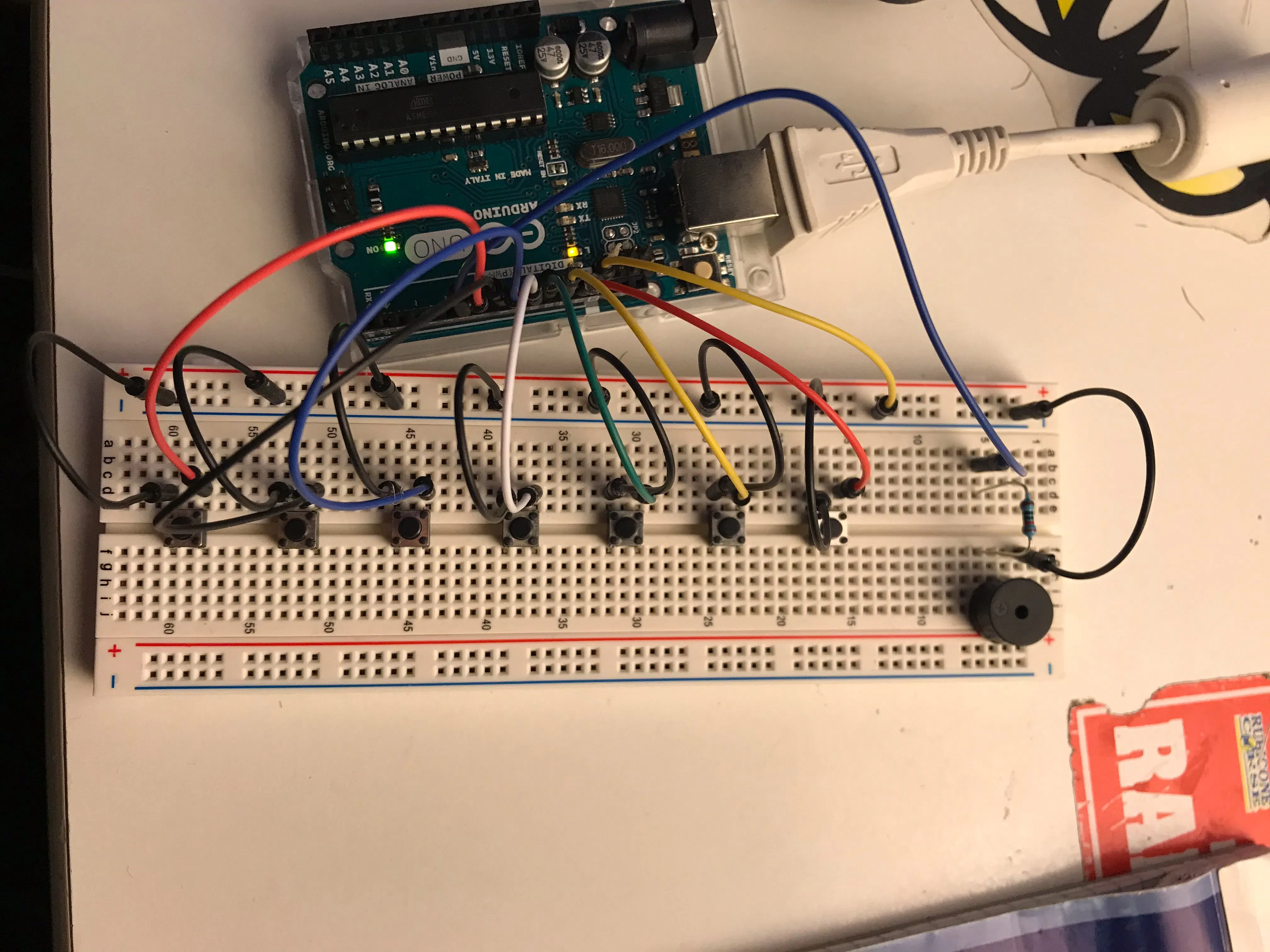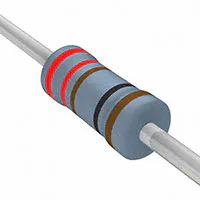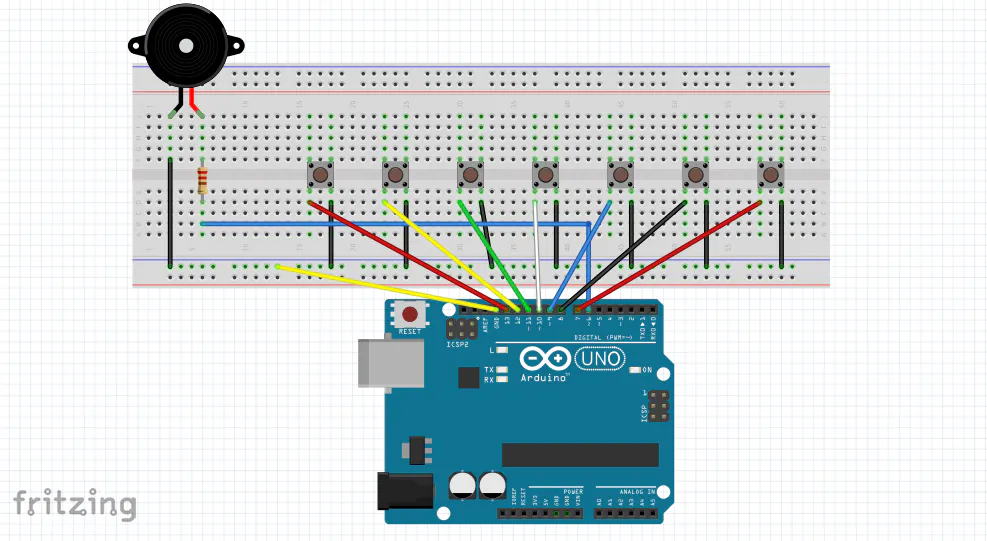# Arduino Keyboard

As a musician and music passionate, this little Keyboard is my first (but not last) Arduino project. Hope you have fun with it :)

BeginnerWork in progress12 minutes26,872## Things used in this project

### Hardware componentsArduino UNO
×1SparkFun Pushbutton switch 12mm
×7
 Passive Buzzer
×1Resistor 221 ohm
×1Jumper wires (generic)
×17UTSOURCE Electronic Parts
×1

## Schematics

### Keyboard schematics

And that's how you should wire it, standing to the code## Code

### Arduino Keyboard code

C/C++
That's the code that will make your keyboard play
```#include "pitches.h" //Notes are stored into this library

int C = 7; //First button on pin 7
int D = 8; //Second button on pin 8
int E = 9; //Third button on pin 9
int F = 10; //Fourth button on pin 10
int G = 11; //Fifth button on pin 11
int A = 12; //Sixth button on pin 12
int B = 13; //Seventh button on pin 13

int c[] = {N_C5}; //Plays C Note
int d[] = {N_D5}; //Plays D Note
int e[] = {N_E5}; //Plays E Note
int f[] = {N_F5}; //Plays F Note
int g[] = {N_G5}; //Plays G Note
int a[] = {N_A5}; //Plays A Note
int b[] = {N_B5}; //Plays B Note

int duration(500); //Every note lasts 500 milliseconds. You can change it as you like

void setup() {
//Set every button as an INPUT_PULLUP
pinMode(C, INPUT_PULLUP);
pinMode(D, INPUT_PULLUP);
pinMode(E, INPUT_PULLUP);
pinMode(F, INPUT_PULLUP);
pinMode(G, INPUT_PULLUP);
pinMode(A, INPUT_PULLUP);
pinMode(B, INPUT_PULLUP);
}

void loop() {
if (digitalRead(C) == LOW) { //Reads button state when pressed
for (int Note = 0; Note < 1; Note++) {
tone(6, c[Note], duration); //Plays note on pin 6, where the Buzzer is connected (change it if you connected it on another pin)
}
}
if (digitalRead(D) == LOW) {
for (int Note = 0; Note < 1; Note++) {
tone(6, d[Note], duration);
}
}
if (digitalRead(E) == LOW) {
for (int Note = 0; Note < 1; Note++) {
tone(6, e[Note], duration);
}
}
if (digitalRead(F) == LOW) {
for (int Note = 0; Note < 1; Note++) {
tone(6, f[Note], duration);
}
}
if (digitalRead(G) == LOW) {
for (int Note = 0; Note < 1; Note++) {
tone(6, g[Note], duration);
}
}
if (digitalRead(A) == LOW) {
for (int Note = 0; Note < 1; Note++) {
tone(6, a[Note], duration);
}
}
if (digitalRead(B) == LOW) {
for (int Note = 0; Note < 1; Note++) {
tone(6, b[Note], duration);
}
}
}
```

## Credits

### Gabriele Scordamaglia

6 projects • 41 followers
23 YO, Automation Engineering student in Italy.